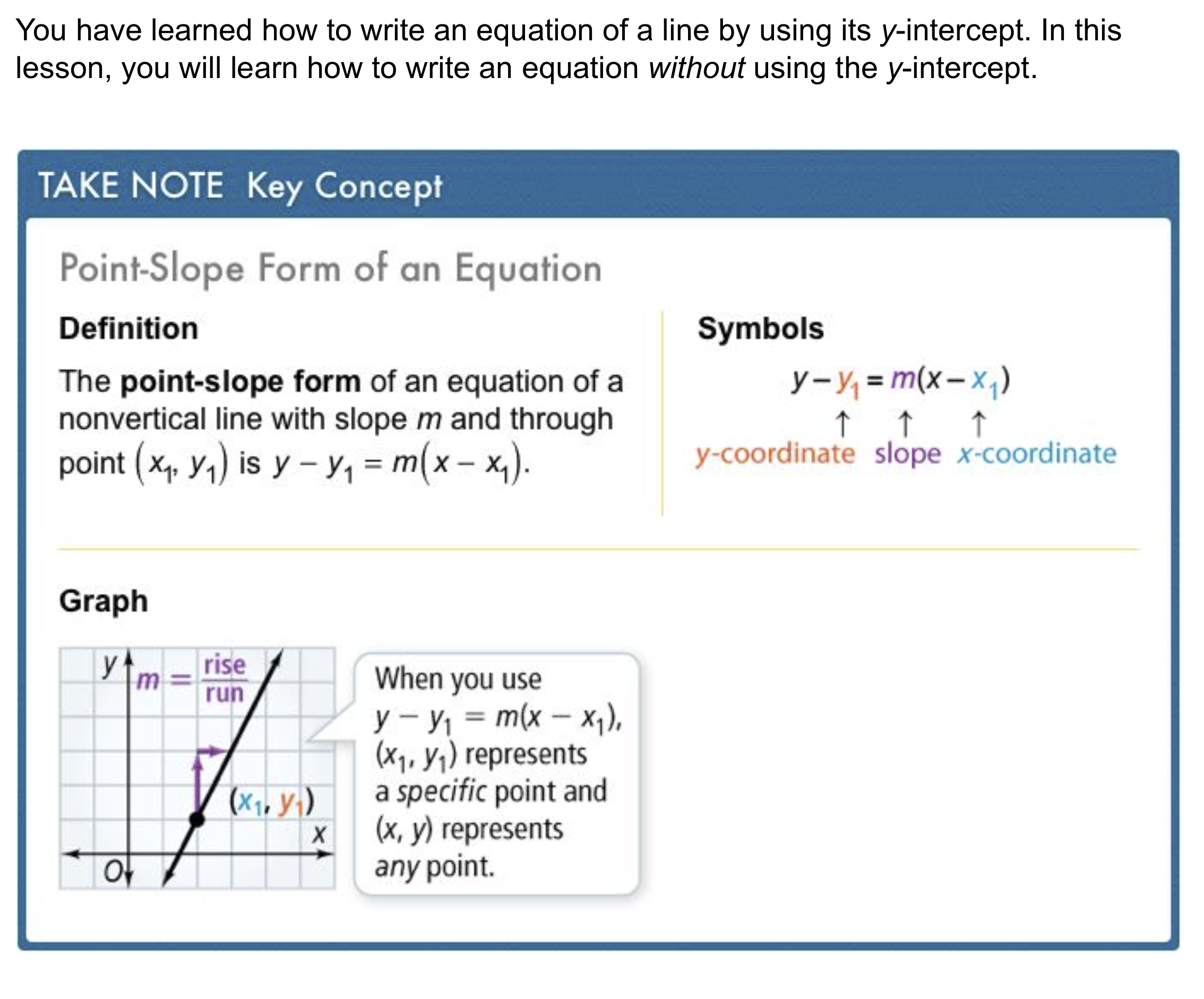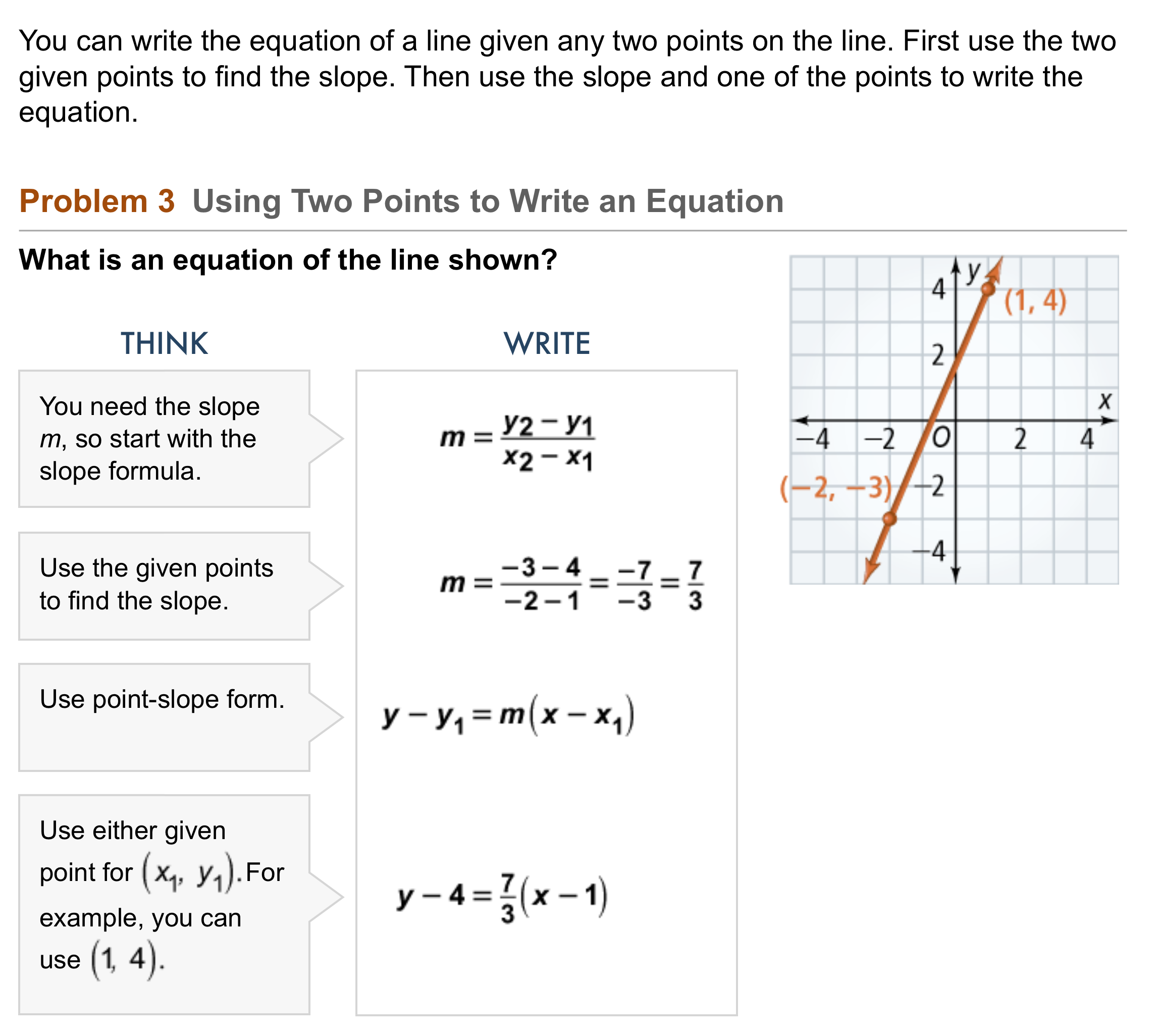Algebra 1 5-4 Guided Practice: Point-Slope Form
starstarstarstarstarstarstarstarstarstar
by Matthew Richardson
| 12 Questions1
1
10
Solve It! The red line shows the altitude of a hot-air balloon during its linear descent. What is an equation of the line in slope-intercept form? Hint: What is the altitude of the balloon when it starts its descent at x = 0?
y = 2x + 650
y = -10x + 640
y = -2x + 650a
2
3
Recall: The general equation used to represent slope-intercept form is:
This format is named slope-intercept form because the equation reveals the slope, m, and y-intercept, b.
2
10
Take Note: What is the general equation used to represent point-slope form ?
Enter the equation as it is shown in the Key Concept box above.
3
10
Take Note: Explain why point-slope form is given that name.4
4
10
Problem 1 Got It?
A
B
C
D
5
10
Problem 2 Got It? What is the graph of the equation?
Be sure to include relevant graph detail: label axes, indicate units on both axes, and use arrows to represent end behavior, as appropriate.6
7
6
10
Problem 3 Got It? In the last step of Problem 3, use the point (-2, -3) instead of (1, 4) to write an equation of the line.
y - 4 = (7/3)(x - 2)
y - 4 = (7/3)(x + 2)
y - 3 = (7/3)(x - 2)
y + 3 = (7/3)(x + 2)
7
10
Problem 3 Got It? Reasoning: Rewrite the equations in Problem 3 and in the previous item in slope-intercept form. Compare the two rewritten equations. What can you conclude?8
9
10
11
8
20
Problem 4 Got It? The table shows the number of gallons of water y in a tank after x hours. The relationship is linear. What is an equation in point-slope form that models the data?
9
10
Problem 4 Got It? Refer to the point-slope form equation you created in the previous item. What does the slope represent?
10
10
Problem 4 Got It? Reasoning: Rewrite the equation from the previous item in slope-intercept form.
11
10
Problem 4 Got It? Reasoning: Refer to the slope-intercept form equation you created in the previous item. What does the y-intercept represent?12
12
10
Take Note: Summarize the content of this lesson.